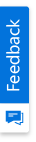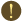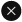We use cookies to give you the best experience on our website. If you continue to browse, then you agree to our privacy policy and cookie policy.Unfortunately, activation email could not send to your email. Please try again.# Change Excel chart series color in C#, VB.NET

Platform: WinForms |
Control: XlsIO

Syncfusion Excel (XlsIO) library is a .NET Excel library used to create, read, and edit Excel documents. Also, converts Excel documents to PDF files. Using this library, you can change Excel chart series color in C#, VB.NET.

Series for which you need to change the color in chart shape has to be accessed and stored in a serie object of IChartSerie interface. Then the data points need to be accessed and foreground color index must be set to change Excel chart series color.

## Steps to change Excel chart series color, programmatically:

Step 1: Create a new C# console application project.Create a new C# console application

Step 2: Install the Syncfusion.XlsIO.WinForms NuGet package as reference to your .NET Framework application from NuGet.org.Install NuGet package

Step 3: Include the following namespace in the Program.cs file.

C#

```using Syncfusion.XlsIO;
```

VB.NET

```Imports Syncfusion.XlsIO
```

Step 4: Use the following code snippet to change Excel chart series color in C#, VB.NET.

C#

```using (ExcelEngine excelEngine = new ExcelEngine())
{
//Instantiate the application object
IApplication application = excelEngine.Excel;

IWorkbook workbook = application.Workbooks.Create(1);

//The first worksheet object in the worksheets collection is accessed
IWorksheet worksheet = workbook.Worksheets;
worksheet.Name = "First Chart";

//Entering the chart data
worksheet.Range["A1"].Text = "Texas books Unit sales";
worksheet.Range["A1:D1"].Merge();
worksheet.Range["A1"].CellStyle.Font.Bold = true;

worksheet.Range["B2"].Text = "Jan";
worksheet.Range["C2"].Text = "Feb";
worksheet.Range["D2"].Text = "Mar";

worksheet.Range["A3"].Text = "Austin";
worksheet.Range["A4"].Text = "Dallas";
worksheet.Range["A5"].Text = "Houston";
worksheet.Range["A6"].Text = "San Antonio";

worksheet.Range["B3"].Number = 53.75;
worksheet.Range["B4"].Number = 52.85;
worksheet.Range["B5"].Number = 59.77;
worksheet.Range["B6"].Number = 96.15;

worksheet.Range["C3"].Number = 79.79;
worksheet.Range["C4"].Number = 59.22;
worksheet.Range["C5"].Number = 10.09;
worksheet.Range["C6"].Number = 73.02;

worksheet.Range["D3"].Number = 26.72;
worksheet.Range["D4"].Number = 33.71;
worksheet.Range["D5"].Number = 45.81;
worksheet.Range["D6"].Number = 12.17;

//Adding a New (Embedded chart) to the worksheet
IChartShape shape = worksheet.Charts.Add();
shape.ChartType = ExcelChartType.Column_Clustered;
shape.DataRange = worksheet.Range["B3:D6"];
shape.IsSeriesInRows = false;
shape.PrimaryCategoryAxis.Title = "City";
shape.PrimaryCategoryAxis.CategoryLabels = worksheet["A3:A6"];
shape.PrimaryValueAxis.Title = "Sales (in Dollars)";
shape.ChartTitle = "Texas Books Unit Sales";
shape.HasDataTable = true;

//Setting the serie names
IChartSerie serieOne = shape.Series;
serieOne.Name = "Jan";
IChartSerie serietwo = shape.Series;
serietwo.Name = "Feb";
IChartSerie seriethree = shape.Series;
seriethree.Name = "March";

//Changing the series color
serietwo.DataPoints.DefaultDataPoint.DataFormat.AreaProperties.ForegroundColorIndex = ExcelKnownColors.Red;

//Save the file
workbook.SaveAs("Output.xlsx");

System.Diagnostics.Process.Start("Output.xlsx");}
```

VB.NET

```Using excelEngine As ExcelEngine = New ExcelEngine()
'Instantiate the application object
Dim application As IApplication = excelEngine.Excel

Dim workbook As IWorkbook = application.Workbooks.Create(1)

'The first worksheet object in the worksheets collection is accessed
Dim worksheet As IWorksheet = workbook.Worksheets(0)
worksheet.Name = "First Chart"

'Entering the chart data
worksheet.Range("A1").Text = "Texas books Unit sales"
worksheet.Range("A1:D1").Merge()
worksheet.Range("A1").CellStyle.Font.Bold = True

worksheet.Range("B2").Text = "Jan"
worksheet.Range("C2").Text = "Feb"
worksheet.Range("D2").Text = "Mar"

worksheet.Range("A3").Text = "Austin"
worksheet.Range("A4").Text = "Dallas"
worksheet.Range("A5").Text = "Houston"
worksheet.Range("A6").Text = "San Antonio"

worksheet.Range("B3").Number = 53.75
worksheet.Range("B4").Number = 52.85
worksheet.Range("B5").Number = 59.77
worksheet.Range("B6").Number = 96.15

worksheet.Range("C3").Number = 79.79
worksheet.Range("C4").Number = 59.22
worksheet.Range("C5").Number = 10.09
worksheet.Range("C6").Number = 73.02

worksheet.Range("D3").Number = 26.72
worksheet.Range("D4").Number = 33.71
worksheet.Range("D5").Number = 45.81
worksheet.Range("D6").Number = 12.17

'Adding a New (Embedded chart) to the Worksheet
Dim shape As IChartShape = worksheet.Charts.Add()
shape.ChartType = ExcelChartType.Column_Clustered
shape.DataRange = worksheet.Range("B3:D6")
shape.IsSeriesInRows = False
shape.PrimaryCategoryAxis.Title = "City"
shape.PrimaryCategoryAxis.CategoryLabels = worksheet("A3:A6")
shape.PrimaryValueAxis.Title = "Sales (in Dollars)"
shape.ChartTitle = "Texas Books Unit Sales"
shape.HasDataTable = True

'Setting the serie names
Dim serieOne As IChartSerie = shape.Series(0)
serieOne.Name = "Jan"
Dim serietwo As IChartSerie = shape.Series(1)
serietwo.Name = "Feb"
Dim seriethree As IChartSerie = shape.Series(2)
seriethree.Name = "March"

'Changing the series color
serietwo.DataPoints.DefaultDataPoint.DataFormat.AreaProperties.ForegroundColorIndex = ExcelKnownColors.Red

'Save the file
workbook.SaveAs("Output.xlsx")

System.Diagnostics.Process.Start("Output.xlsx")
End Using
```

A complete Windows Forms working example of how to change Excel chart series color in C# and VB can be downloaded from Change Excel Chart Series Color.zip.

By executing the program, you will get the output Excel file as shown below.Output Excel document

Refer here to explore the rich set of Syncfusion Excel (XlsIO) library features.

How to change the color of single Data point in XlsIO chart?

How to apply or change colors in Excel pie chart?

How can I change the font color of the title in Chart?

How to set gap width to chart series?

How to split the series of pie of pie chart using XlsIO?

How to filter Excel chart series in C#, VB.NET?

How to set trendlines for Excel chart series in C#, VB.NET?

Note:

Starting with v16.2.0.x, if you reference Syncfusion assemblies from trial setup or from the NuGet feed, include a license key in your projects. Refer the link to learn about generating and registering Syncfusion license key in your application to use the components without trail message.

2X faster development

The ultimate WinForms UI toolkit to boost your development speed.You are using an outdated version of Internet Explorer that may not display all features of this and other websites. Upgrade to Internet Explorer 8 or newer for a better experience.